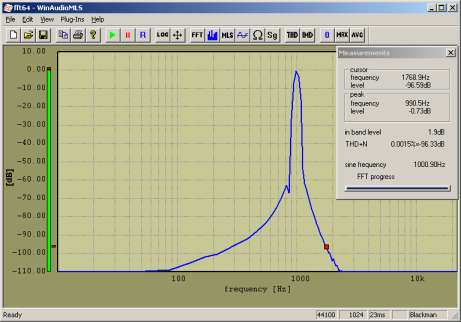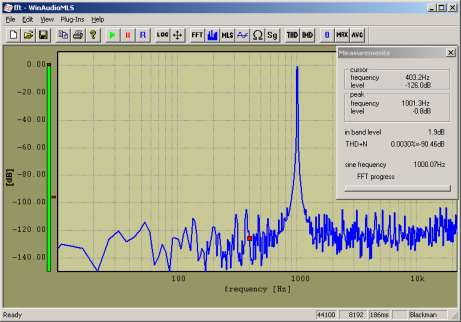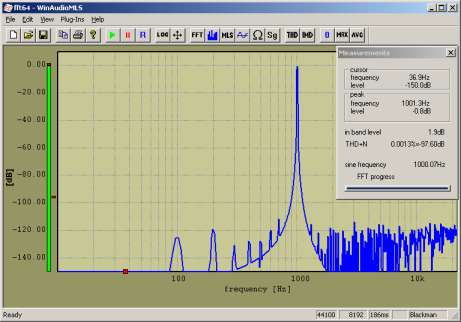# Why 64 bits?

Most audio is recorded with 16 bits resolution. WinAudioMLS uses in the standard mode 32-bit floating point variables to store each sample. From a first look this headroom seems to be more than sufficient. Floating point variables have a dynamic range of more than 200dB which is far much more than any audio format.
But a 32-bit floating point number consists of a 24-bit mantissa and a 8-bit exponent. Each operation of two floating point numbers reduces the available resolution. For a 4096 points FFT there are 12 sequential stages of additions. For a 4096 points FFT the noise introduced by 32 bits floating point operation exceeds the 16-bit quantization noise.

You can easily check this effect. If you analyze an ideal 16-bit sine file with a 1024 points FFT, you get a THD+N close to the theoretical value (97dB).
If you increase the FFT size, both the frequency resolution and the FFT noise increase. For a 8192 points FFT the maximum displayed THD+N is around 90dB.
If you measure in the area above 90dB THD+N for high precision test sets we recommend our 64-bits FFT. This FFT with double precision has a noise floor 144dB below the 32-bits FFT. The CPU load for this FFT is 30% higher. It allows you to measure very low noise even at high frequency resolutions. This plug-in can be easily activated or deactivated.

Below you will find some measurement examples demonstrating the advantages of the 64-bit FFT.
The input signal is a file with an ideal 1kHz sinus sampled with 16-bit.
It can be easily seen that the noise introduced by the 32-bit FFT at 8192 exceeds the quantization  noise and therefore degrades the THD+N measurement by 6dB.

## FFT size 1024 32-bit FFT THD+N 96dB## FFT size 1024 64-bit FFT THD+N 96dB## FFT size 8192 32-bit FFT THD+N 90dB## FFT size 8192 64-bit FFT THD+N 97dB